32 Patterns
MatchQ tests whether something matches a pattern.
{a, x, b} matches the pattern {_, x, _}:
 In:=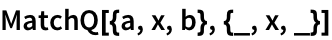Out=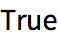{a, b, c} doesnt match, because it has b in the middle, rather than x:
 In:=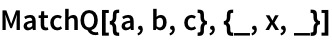Out=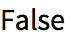Any list with two elements matches the pattern {_, _}:
 In:=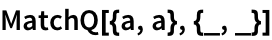Out=A list with three elements doesnt match the pattern {_, _}:
 In:=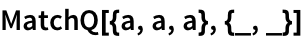Out=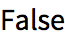MatchQ lets you test one thing at a time against a pattern. Cases lets you pick out all the elements (cases) in a list that match a particular pattern.
Find all elements that match the pattern {_, _}:
 In:=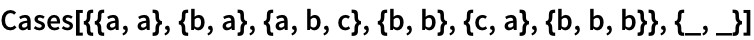Out=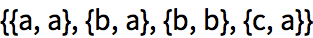Find all elements that match {b, _} (i.e. cases of b followed by something):
 In:=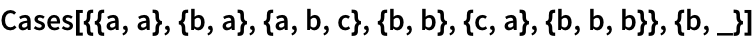Out=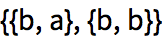This is what you get if you test whether each element matches {b, _}:
 In:=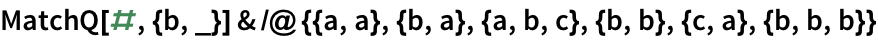Out=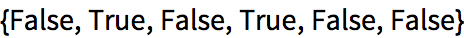Using Select to select what matches gives the same result as Cases:
 In:=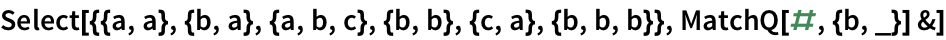Out=Find all cases of either a or b, followed by something:
 In:=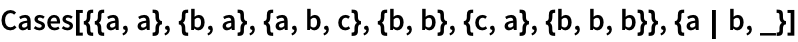Out=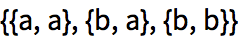Lets look at another example, based on creating a list, then picking out elements that match particular patterns.
Create a list by getting the digits of a range of numbers:
 In:=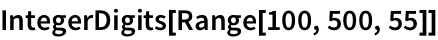Out=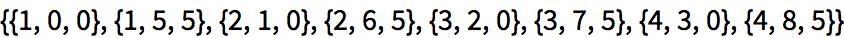Find cases that end in 5:
 In:=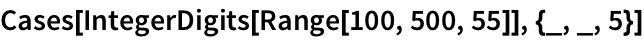Out=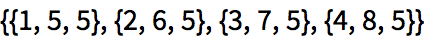Find cases with 1 or 2 in the middle:
 In:=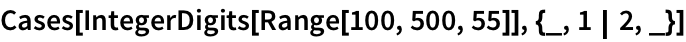Out=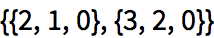The notation __ (double blank) in a pattern indicates any sequence of things.
Find cases consisting of any sequence, ending with b:
 In:=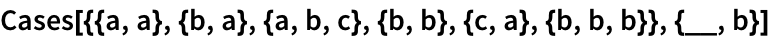Out=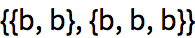Find sequences ending with a or b, or beginning with c:
 In:=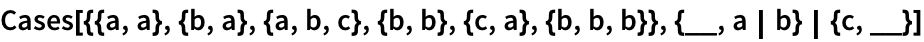Out=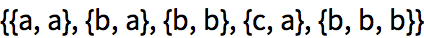Patterns arent just about lists; they can involve anything.
Pick out cases that match the pattern f[_]:
 In:=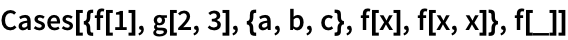Out=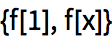One of the many uses of patterns is to define replacements. /. (slash dot) performs a replacement.
Replace b with Red in a list:
 In:=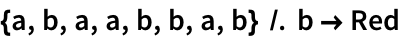Out=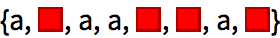In:=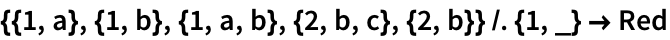Out=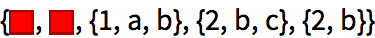You can give a list of replacements to use:
 In:=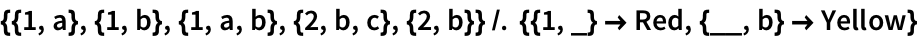Out=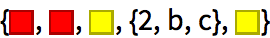The blank pattern _ matches absolutely anything. This means, for example, that {_,_} matches any list of two elements. But what if you want to insist that the two elements be the same? You can do that using a pattern like {x_,x_}.
{_, _} matches any list of two elements, whether the elements are the same or not:
 In:=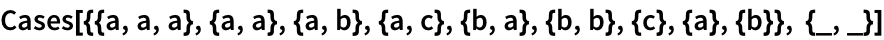Out=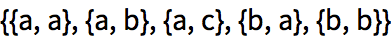{x_, x_} matches only lists of two identical elements:
 In:=Out=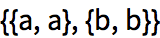x_ is an example of a named pattern. Named patterns are especially important in replacements, because they give one way to make use of parts of what ones replacing.
Use the named pattern x_ in a replacement:
 In:=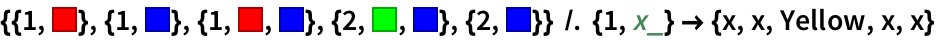Out=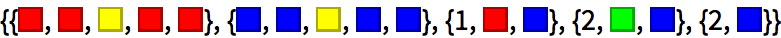The form ab is usually called a rule. If x_ appears on the left-hand side of a rule, then whatever the x_ matches can be referred to on the right-hand side as x.
Use x in the right-hand side of the rule to refer to what x_ matches:
 In:=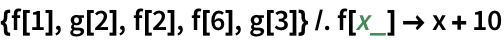Out=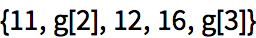You can use rules inside Cases as well.
Pick out elements in the list that match f[x_], and give the result of replacing them by x+10:
 In:=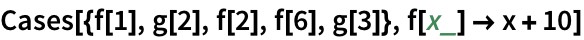Out=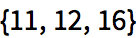Later on, well see how named patterns are crucial to defining your own functions in the Wolfram Language.
 _ pattern standing for anything (“blank”) __ pattern standing for any sequence (“double blank”) x_ pattern named x a|b pattern matching a or b MatchQ[expr,pattern] test whether expr matches a pattern Cases[list,pattern] find cases of a pattern in a list lhsrhs rule for transforming lhs into rhs expr/.lhsrhs replace lhs by rhs in expr
32.1Find lists of length 3 or more beginning with 1 and ending with 9 in IntegerDigits[Range]»
Expected output:
 Out[]=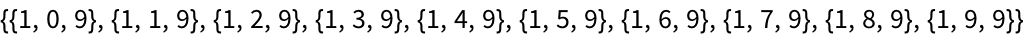Answer & check your solution
32.2Find lists of three identical elements in IntegerDigits[Range]»
Expected output:
 Out[]=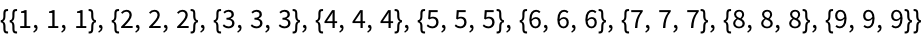Answer & check your solution
32.3In the digit lists for the first 1000 squares, find those that begin with 9 and end with 0 or 1. »
Expected output:
 Out[]=Answer & check your solution
32.4In IntegerDigits[Range] replace all 0s by Gray and all 9s by Orange»
Expected output:
 Out[]=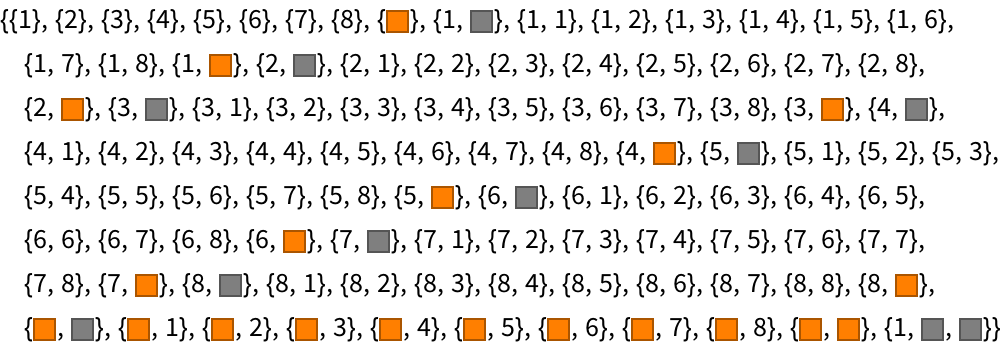Answer & check your solution
32.5Make a list of the digits of 2^1000, replacing all zeros by Red»
Expected output:
 Out[]=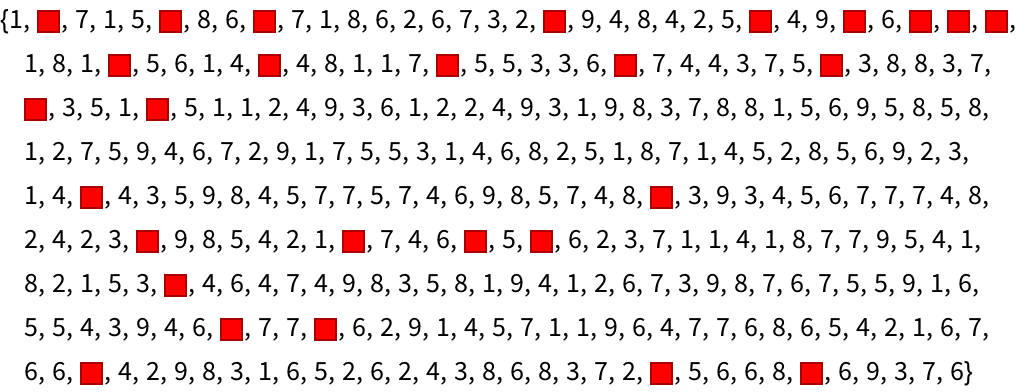Answer & check your solution
32.6Remove the vowels a, e, i, o and u from the list of characters in The Wolfram Language»
Expected output:
 Out[]=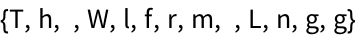Answer & check your solution
32.7Find a simpler form for Select[IntegerDigits[2^1000], #0||#1&]»
Expected output:
 Out[]=Answer & check your solution
32.8Find a simpler form for Select[IntegerDigits[Range[100, 999]], First[#]Last[#]&]»
Expected output:
 Out[]=Answer & check your solution
No. They just have to be consistent inside a given pattern. Different patterns can reuse the same name for different purposes, and the name can also appear outside the pattern.
What else can be used to define patterns?
Well discuss several more things in Section 41.
Whats the difference between | and ||?
p|q is a pattern construct, that matches either p or q. p||q is a logic construct, that tests whether p or q is True.
How is /. interpreted?
Its the function ReplaceAll. Replace tries to replace a whole expression. ReplaceList gives a list of results from all possible ways to match a particular pattern.
If /. has several replacements, which one will it use?
It uses the first one that applies. If replacements apply to multiple levels of an expression, /. will use it on the outermost level.